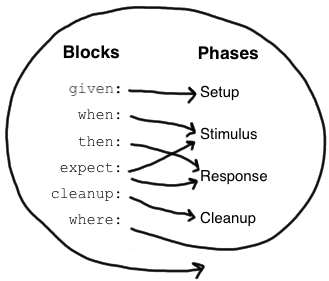• Setup 初始化
• Stimulus 刺激
• Response 响应
• Cleanup 清理资源

## 块

### Given 块

``````given:
def stack = new Stack()
def elem = "push me"
``````

### When 和 Then 块

when 和 then 块始终一起出现。他们描述了刺激和预期的反应。

``````when:   // 刺激
then:   // 响应
``````

``````when:
stack.push(elem)

then:
!stack.empty
stack.size() == 1
stack.peek() == elem
``````

### Expect 块

``````when:
def x = Math.max(1, 2)

then:
x == 2
``````
``````expect:
Math.max(1, 2) == 2
``````

### Cleanup 块

``````given:
def file = new File("/some/path")
file.createNewFile()

// ...

cleanup:
file.delete()
``````

### Where 块

``````def "computing the maximum of two numbers"() {
expect:
Math.max(a, b) == c

where:
a << [5, 3]
b << [1, 9]
c << [5, 9]
}
``````

``````def "computing the maximum of two numbers"() {
expect:
Math.max(a, b) == c

where:
a | b | c
5 | 1 | 5
3 | 9 | 9
}
``````